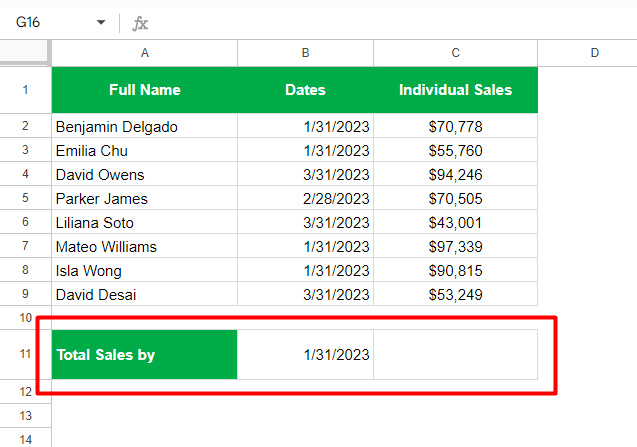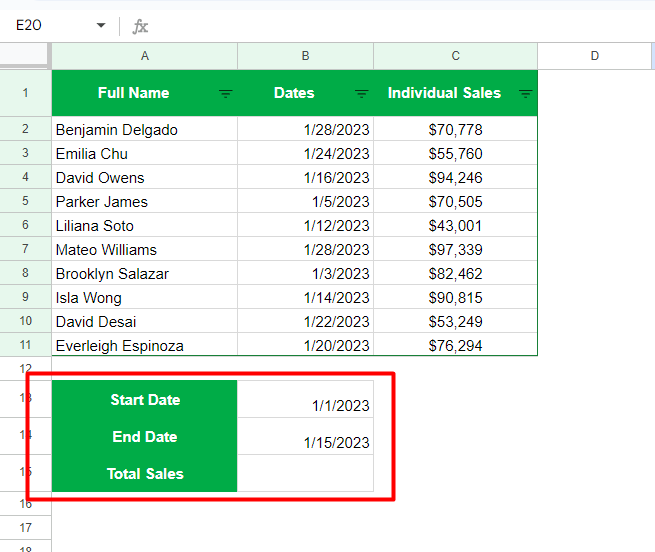# SUMIFS & SUMIF with Date Range in Google Sheets

The SUMIF and SUMIFS are powerful functions in Google Sheets to get the sum of cell values between two or more date ranges. They calculate the sum of a range of values satisfying the criteria defined by the users.

You may wish to calculate the total amount spent within a given date range or determine the total number of orders received.

In this case, the SUMIF is the best choice.

So if you seek a solution to calculate a certain number within a given date range, the solution is the use of SUMIFS and SUMIF with Date Range in Google Sheets.

This article is a step-by-step guide to finding the sum of cell values using the SUMIF and SUMIFS functions between two or more date ranges. Simply put, we will discuss using these functions with a date range as the criteria.

Now, without any further ado, let’s begin.

Calculating the sum of cell values between given date ranges is quite hard, so I suggest downloading the following Google Sheet with the data we will use to demonstrate the SUMIF and SUMIFS functions in this article.

## Explained: The SUMIF function in Google Sheets

The SUMIF is a simple function in Google Sheets that helps you find the sum of cell values between two date ranges.

The general syntax is as follows,

``=SUMIF(range, criterion, [sum_range])``

Each argument in the above formula must be replaced with the proper information.

• range” – This argument must be replaced with the group of cells containing dates. It will be tested against the criteria defined by you.
• criteria” – Here, you need to define the criteria or the date range for which you wish to find the sum of cell values.
• sum_range” – It is the range to be summed. The function calculates the sum in this range, for example, the amount spent, sales, number of orders, etc.

Let’s consider a simple example below where we wish to calculate the total sales made by our sales rep in January 2023.

The final formula using the SUMIF will look something like below,

=SUMIF(B2:B9,B11,C2:C9)

• The “B2:B9” is the “range” here against which our criteria (sales made in January 2023) will be tested
• The “B11” represents the “criteria” as sales made by the individual sales rep in January 2023 (31-Jan-2023)
• And the “C2:C9” is the “sum_range”, which is summed against the criteria

## How to Use SUMIF for a Given Date Range in Google Sheets

The SUMIF function is useful for a single criterion or condition, as the above example discusses.

Task: Calculating the total sales in January 2023.

Let us see the steps to use the SUMIF function in Google Sheets.

• Open the Google Sheet and go to the spreadsheet containing the data table
• Create a new table as shown belowEnter this Formula: `=SUMIF(B2:B9,B11,C2:C9)`

Follow the remaining steps below to type this formula if you don’t want to copy and paste it.

• Click on the cell “C11” as we wish to calculate the sum of the cells in that cell
• Type “=sumif
• Select the first option from the popup or pressTab” on your keyboard
• Now, for “range”, let us select the dates column by putting “B2:B9” as our criteria will be tested against the entries in this column
• Press “,” on your keyboard to move to the next argument
• For “criteria”, as we plan to find the total sales in January 2023, either we can manually type the date “1/31/2023” or select the cell reference value as “B11
• Press “,
• Next, we need to select the “sum_range” by putting “C2:C9” as we have sales made by individual executives available in column C
• Close the brackets using “)

The final formula should look like this:

``=SUMIF(B2:B9,B11,C2:C9)``

Google Sheets will instantly calculate the total sales in January 2023 at \$314,692.

## Explained: The SUMIFS function in Google Sheets

In contrast to the SUMIF function, which lets you find the sum of cell values for a single criterion, the SUMIFS function allows you to use multiple criteria or conditions.

It is an array formula that is quite hard to use. But it all boils down to your understanding of the criteria (two or more date ranges to use) and knowledge of the function.

The syntax for the SUMIFS function in Google Sheets is as follows,

``=SUMIFS(sum_range, criteria_range1, criteria1, [criteria_range2, criteria2], …)``

The arguments for this formula are very similar to the one we discussed above for the SUMIF function.

• sum_range” – It represents the range of cells to be summed. You may wish to calculate the amount spent, sales, number of orders, etc.
• criteria_range1” – This argument needs to be replaced with the group of cells against which your first criteria will be tested
• criteria1” – Here, you can define your first criteria to get the sum of the cells
• criteria_range2” – It is for the second group of cells against which your second criteria will be tested
• criteria2” – It is for defining the second criteria with which you wish to find the sum of the cells

You can define any number of criteria or conditions to get the sum of cells using the SUMIF function. There is no limit, and it depends upon the size of your dataset.

## How to Use SUMIFS between Two or More Date Ranges

The SUMIFS is a powerful array formula capable of performing multiple calculations based on the criteria defined by the users.

It scans the entire column provided to return the values that match the defined criteria or conditions.

We are about to use two comparison or logical operators in Google Sheets,

• Greater Than or Equal To – “>=
• Less Than or Equal To – “<=

These operators compare the cell values against the criteria defined by us. In simple words, the “>=” operator ensures values that are greater than the given date, and the “<=” operator ensures values that are less than or equal to the given date.

Task: Calculate the total sales made by the sales reps between January 1, 2023, to January 15, 2023.

Let’s begin,

• Open the Google Sheet and go to the spreadsheet containing the data table
• Create a new table as shown belowTo get the Total Sales, enter this formula in cell B15:

``=SUMIFS(C2:C11,B2:B11,">="&B13,B2:B11,"<="&B14)``

If you don’t want to copy and paste the formula, below is a breakdown of how to type it.

• Click on the cell “B15”, where we wish to calculate the sum of the cells
• Type “=sumifs
• Select the first option from the popup or pressTab” on your keyboard
• For the “sum_range”, let us choose “C2:C11” as the sales made by an individual representative are available in Column C
• Press “,” on your keyboard to move to the next argument
• Now, for “criteria_range1”, we will choose the dates column from B2 to B11 and put “B2:B11
• Press “,
• Next, for “criteria1”, you must start with the Greater Than or Equal To operator as “>=”
(Make sure to place this operator between quotation marks “”, otherwise you will get an error at the end)
• Then, type “&” and put the first criteria, which in this case is the date “1/1/2023” (January 1, 2023). Here, you can either manually type the date or select the cell reference from our new table as “B13”. Refer to the following image
• Press “,
• For the second range, “criteria_range2”, we will choose the same date column as “B2:B11
• Press”,
• The second criterion will start with Less Than or Equal To operator as “<=”
(Make sure you put the operator in quotation marks “”)
• Type “&” and put the end date. We will choose the cell reference from our new table as “B14
• Complete the brackets using “)
• Press Enter” on your keyboard

The above steps are for SUMIFS between Two Date Ranges. However, you can repeat the process from steps 8 to 11 for any number of date ranges you wish.

There is no limit; you can define multiple criteria and conditions to calculate the sum of cell values.

After using our Example Google Sheet and following the steps above, the final formula should look like below.

``=SUMIFS(C2:C11,B2:B11,">="&B13,B2:B11,"<="&B14)``

Precaution: For the SUMIFS function to work in Google Sheets, ensure that the “criteria_range” argument is equally sized for all criteria or conditions. It should also match the size of the “sum_range” argument.

To validate the results, you can use the filters by hovering over the toolbar section of the main menu and clicking on the Filter icon, as shown below.

Filter the table by Column B by choosing the option “Sort A to Z” which will sort the column in ascending order (newest to oldest).

Now, select the first four cells of column C that represent the sales before January 15, 2023. You can see the sum of these cells at the lower-rightmost section of the screen. Refer to the image below,

## Final Words on Using SUMIFS & SUMIF with Date Range in Google Sheets

That’s it.

Both SUMIF and SUMIFS are highly useful functions by Google Sheets. They are particularly useful for financing, e-commerce data management, budgeting, etc.

Let us know in the comments below if you are stuck somewhere or facing any difficulties using these formulas. Feel free to comment below because I answer all the questions on our blogs.

I hope this article helped you learn the SUMIF and SUMIFS functions for calculating the sum of cell values between given date ranges.